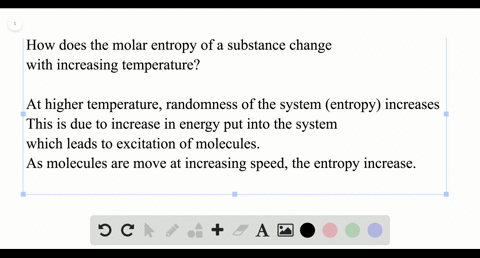### How does the molar entropy of a substance change …

01:10
LP
Problem 48

Predict the conditions (high temperature, low temperature, all temperatures, or no temperatures) under which each reaction is spontaneous.
\begin{equation}\begin{array}{l}{\text { a. } \mathrm{H}_{2} \mathrm{O}(g) \longrightarrow \mathrm{H}_{2} \mathrm{O}(l)} \\ {\text { b. } \mathrm{CO}_{2}(s) \longrightarrow \mathrm{CO}_{2}(g)} \\ {\text { c. } \mathrm{H}_{2}(g) \longrightarrow 2 \mathrm{H}(g)} \\ {\text { d. } 2 \mathrm\ {NO}_{2}(g) \longrightarrow 2 \mathrm\ {NO}(g)+\mathrm{O}_{2}(g) \text { (endothermic) }}\end{array}
\end{equation}

PART A: low temperatures
PART B: high temperatures
PART C: high temperatures
PART D: high temperatures

## Discussion

You must be signed in to discuss.

## Video Transcript

So this question is willing you to figure out at what temperature range are these reactions spontaneous? So for question party, from this reaction, we can calculate Don't h end of the s of reaction understanding state and see what Delta G would be. And now that determine what our temperature range could be. So I'm gonna go delta H by doing, um, don't h formation of products minus reactant ce and so you can find these in your textbook. So for the liquid is to 85 points, eight minus negative to 41 point eight for the gas. And that gives you minus 44. So it gives you a negative number, then calculate Death s if you want to. But you could also just look at it intuitively and see that you're going from a gas to a liquid. So you're changing and chippy is gonna decrease. Could become because you're becoming more ordered. So if your delta age is negative and you're dealt s is negative. Don't g she caused at the h minus t don't s you have and you won't tell Taji to be as negative as possible. So the H is negative in Delta s is negative, which means this number overall was gonna be a positive number. So you won't tear to be a smallest possible. So the this don't The h is is negative, as is larger than this value, so you could mean more negative. So this is spontaneous at a lot of temperature at low T. So for the next one, calculate dealt a very similar way Amiga panties in your textbook or online. You're just doing products finest reactant again and you get 1000 of H. And then if you look at how this reaction is going was it's going from I saw it too. Gases becoming more disorder, too. Don't s also positive. So now you have Delta G, which is equal to a positive number minus another positive number, and you won't tell T to be negative. So you want t to be a CZ? Largest possible. So this spontaneous at large T let's do a up there to show the case that is large. All right, let's bring in the table again just for real quick. So for part C, averaging gas, going 2 to 8 so you can't let this again. But she and account for the Moler coefficient. How many moles of hydrogen is there that serves to So you do two times your don't age of products, which to 18 minus zero. So I give you positive number, and then you can calculate Delta s the same way, or you can look at it and say You're going from one gas molecules Mole 22 mo's of gas molecules. It's that's becoming more disordered. So there's something positive. And so this is the same as a previous case where you had a positive positive. So this is that we'll be spontaneous at high temperature, a high tea. Lastly, we have party and they tell you this reactions and author mixed. We don't need to calculate anything. I mean, Delta H. It's positive since, and the thermic and the system is gaining heat. I'm dealt s You're going from two moles of gas. The three miles of the gas is becoming more disordered, so entry is also increasing. So again you have the same situation. So this spontaneous at large tea as well